# Wine quality prediction with L1 regression#

# install dependencies and select solver
%pip install -q amplpy matplotlib

SOLVER = "highs"

from amplpy import AMPL, ampl_notebook

ampl = ampl_notebook(
modules=["highs"],  # modules to install
)  # instantiate AMPL object and register magics

     ━━━━━━━━━━━━━━━━━━━━━━━━━━━━━━━━━━━━━━━━ 5.6/5.6 MB 14.7 MB/s eta 0:00:00
?25hUsing default Community Edition License for Colab. Get yours at: https://ampl.com/ce
Licensed to AMPL Community Edition License for the AMPL Model Colaboratory (https://colab.ampl.com).


## Problem description#

Regression is the task of fitting a model to data. If things go well, the model might provide useful predictions in response to new data. This notebook shows how linear programming and least absolute deviation (LAD) regression can be used to create a linear model for predicting wine quality based on physical and chemical properties. The example uses a well known data set from the machine learning community.

Physical, chemical, and sensory quality properties were collected for a large number of red and white wines produced in the Portugal then donated to the UCI machine learning repository (Cortez, Paulo, Cerdeira, A., Almeida, F., Matos, T. & Reis, J.. (2009). Wine Quality. UCI Machine Learning Repository.) The following cell reads the data for red wines directly from the UCI machine learning repository.

Cortez, P., Cerdeira, A., Almeida, F., Matos, T., & Reis, J. (2009). Modeling wine preferences by data mining from physicochemical properties. Decision support systems, 47(4), 547-553. https://doi.org/10.1016/j.dss.2009.05.016

import pandas as pd
import matplotlib.pyplot as plt
import numpy as np

"https://archive.ics.uci.edu/ml/machine-learning-databases/wine-quality/winequality-red.csv",
sep=";",
)
display(wines)

fixed acidity volatile acidity citric acid residual sugar chlorides free sulfur dioxide total sulfur dioxide density pH sulphates alcohol quality
0 7.4 0.700 0.00 1.9 0.076 11.0 34.0 0.99780 3.51 0.56 9.4 5
1 7.8 0.880 0.00 2.6 0.098 25.0 67.0 0.99680 3.20 0.68 9.8 5
2 7.8 0.760 0.04 2.3 0.092 15.0 54.0 0.99700 3.26 0.65 9.8 5
3 11.2 0.280 0.56 1.9 0.075 17.0 60.0 0.99800 3.16 0.58 9.8 6
4 7.4 0.700 0.00 1.9 0.076 11.0 34.0 0.99780 3.51 0.56 9.4 5
... ... ... ... ... ... ... ... ... ... ... ... ...
1594 6.2 0.600 0.08 2.0 0.090 32.0 44.0 0.99490 3.45 0.58 10.5 5
1595 5.9 0.550 0.10 2.2 0.062 39.0 51.0 0.99512 3.52 0.76 11.2 6
1596 6.3 0.510 0.13 2.3 0.076 29.0 40.0 0.99574 3.42 0.75 11.0 6
1597 5.9 0.645 0.12 2.0 0.075 32.0 44.0 0.99547 3.57 0.71 10.2 5
1598 6.0 0.310 0.47 3.6 0.067 18.0 42.0 0.99549 3.39 0.66 11.0 6

1599 rows × 12 columns

## Model objective: Mean Absolute Deviation (MAD)#

Given $$n$$ repeated observations of a response variable $$y_i$$ (in this wine quality), the mean absolute deviation (MAD) of $$y_i$$ from the mean value $$\bar{y}$$ is

$\text{MAD}\,(y) = \frac{1}{n} \sum_{i=1}^n | y_i - \bar{y}|$
def MAD(df):
return (df - df.mean()).abs().mean()

fig, ax = plt.subplots(figsize=(12, 4))
ax = wines["quality"].plot(alpha=0.6, title="wine quality")
ax.axhline(wines["quality"].mean(), color="r", ls="--", lw=3)

ax.set_xlabel("observation")

plt.show()

MAD =  0.6831779242889846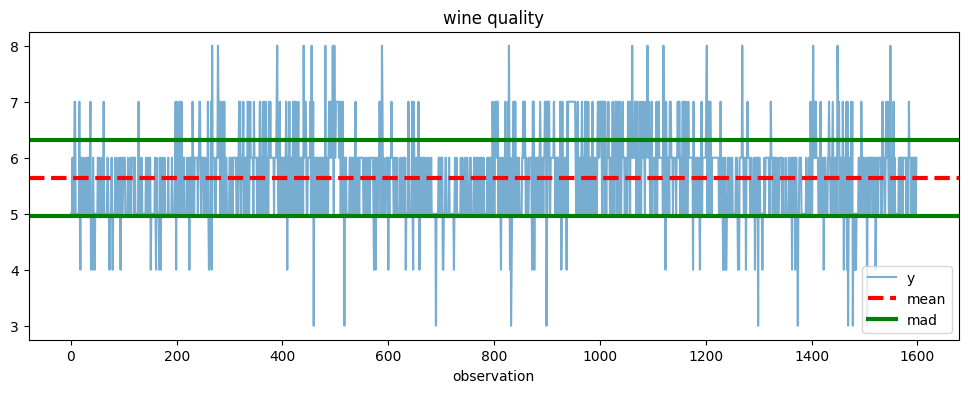## A preliminary look at the data#

The data consists of 1,599 measurements of eleven physical and chemical characteristics plus an integer measure of sensory quality recorded on a scale from 3 to 8. Histograms provides insight into the values and variability of the data set.

fig, axes = plt.subplots(3, 4, figsize=(12, 8), sharey=True)

for ax, column in zip(axes.flatten(), wines.columns):
wines[column].hist(ax=ax, bins=30)
ax.axvline(wines[column].mean(), color="r", label="mean")
ax.set_title(column)
ax.legend()

plt.tight_layout()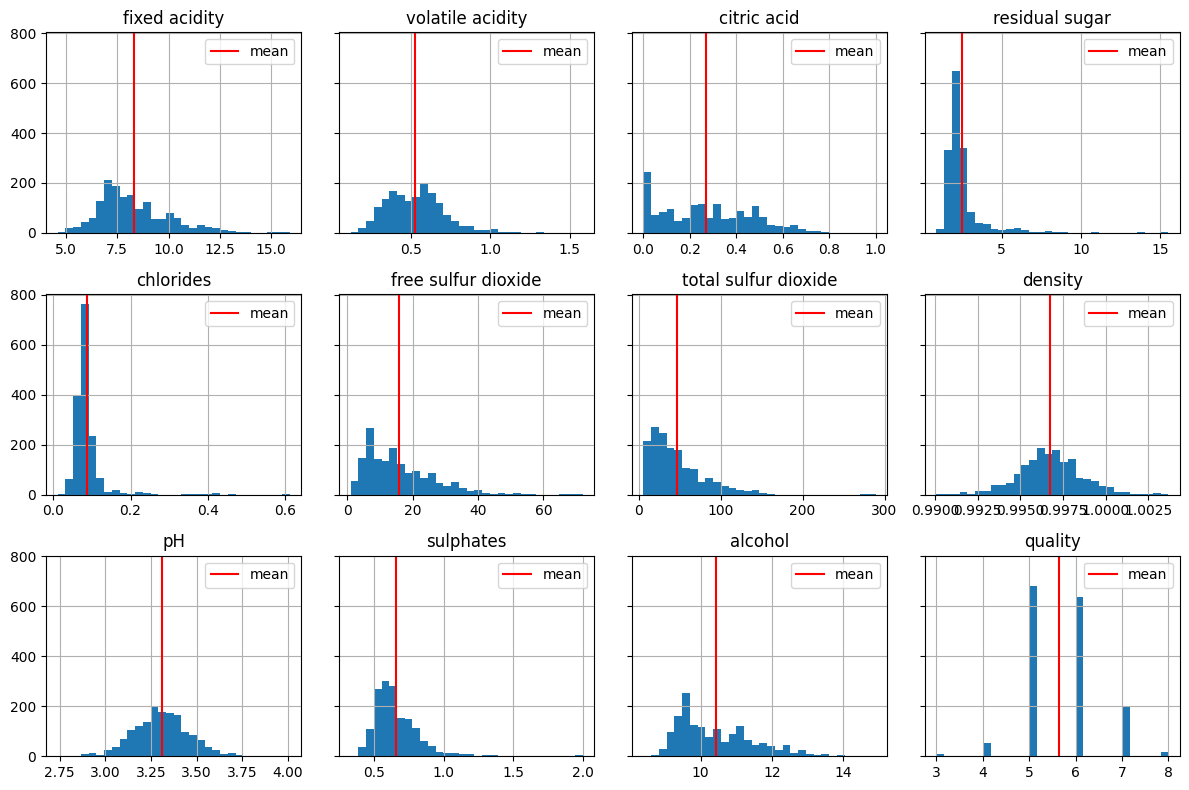## Which features influence reported wine quality?#

The art of regression is to identify the features that have explanatory value for a response of interest. This is where a person with deep knowledge of an application area, in this case an experienced onenologist will have a head start compared to the naive data scientist. In the absence of the experience, we proceed by examining the correlation among the variables in the data set.

_ = wines.corr()["quality"].plot(kind="bar", grid=True)wines[["volatile acidity", "density", "alcohol", "quality"]].corr()

volatile acidity density alcohol quality
volatile acidity 1.000000 0.022026 -0.202288 -0.390558
density 0.022026 1.000000 -0.496180 -0.174919
alcohol -0.202288 -0.496180 1.000000 0.476166
quality -0.390558 -0.174919 0.476166 1.000000

Collectively, these figures suggest alcohol is a strong correlate of quality, and several additional factors as candidates for explanatory variables..

## LAD line fitting to identify features#

An alternative approach is perform a series of single feature LAD regressions to determine which features have the largest impact on reducing the mean absolute deviations in the residuals.

\begin{align*} \min \frac{1}{I} \sum_{i\in I} \left| y_i - a x_i - b \right| \end{align*}

This computation has been presented in a prior notebook.

%%writefile lad_fit_1.mod

set I;

param y{I};
param X{I};

var a;
var b;

var e_pos{I} >= 0;
var e_neg{I} >= 0;

var prediction{i in I} = a * X[i] + b;
s.t. prediction_error{i in I}: e_pos[i] - e_neg[i] == prediction[i] - y[i];

minimize mean_absolute_deviation: sum{i in I}(e_pos[i] + e_neg[i]) / card(I);

Writing lad_fit_1.mod

def lad_fit_1(df, y_col, x_col):
m = AMPL()

m.set["I"] = df.index.values

m.param["y"] = df[y_col]
m.param["X"] = df[x_col]

m.option["solver"] = SOLVER
m.solve()

return m

print(
f'The mean absolute deviation for a single-feature regression is {m.obj["mean_absolute_deviation"].value():0.5f}'
)

HiGHS 1.5.3: HiGHS 1.5.3: optimal solution; objective 0.5411666021
1578 simplex iterations
0 barrier iterations

The mean absolute deviation for a single-feature regression is 0.54117


This calculation is performed for all variables to determine which variables are the best candidates to explain deviations in wine quality.

mad = (wines["alcohol"] - wines["alcohol"].mean()).abs().mean()
vars = {}
for i in wines.columns:
vars[i] = m.obj["mean_absolute_deviation"].value()

fig, ax = plt.subplots()
pd.Series(vars).plot(kind="bar", ax=ax, grid=True)
plt.show()

HiGHS 1.5.3: HiGHS 1.5.3: optimal solution; objective 0.6579111945
1494 simplex iterations
0 barrier iterations

HiGHS 1.5.3: HiGHS 1.5.3: optimal solution; objective 0.5980365332
1642 simplex iterations
0 barrier iterations

HiGHS 1.5.3: HiGHS 1.5.3: optimal solution; objective 0.6457762063
1584 simplex iterations
0 barrier iterations

HiGHS 1.5.3: HiGHS 1.5.3: optimal solution; objective 0.6579111945
1508 simplex iterations
0 barrier iterations

HiGHS 1.5.3: HiGHS 1.5.3: optimal solution; objective 0.6517416082
1628 simplex iterations
0 barrier iterations

HiGHS 1.5.3: HiGHS 1.5.3: optimal solution; objective 0.6579111945
1543 simplex iterations
0 barrier iterations

HiGHS 1.5.3: HiGHS 1.5.3: optimal solution; objective 0.6172771025
1706 simplex iterations
0 barrier iterations

HiGHS 1.5.3: HiGHS 1.5.3: optimal solution; objective 0.6544712022
1648 simplex iterations
0 barrier iterations

HiGHS 1.5.3: HiGHS 1.5.3: optimal solution; objective 0.6579111945
1489 simplex iterations
0 barrier iterations

HiGHS 1.5.3: HiGHS 1.5.3: optimal solution; objective 0.6320777409
1636 simplex iterations
0 barrier iterations

HiGHS 1.5.3: HiGHS 1.5.3: optimal solution; objective 0.5411666021
1578 simplex iterations
0 barrier iterations

HiGHS 1.5.3: HiGHS 1.5.3: optimal solution; objective 0
2 simplex iterations
0 barrier iterations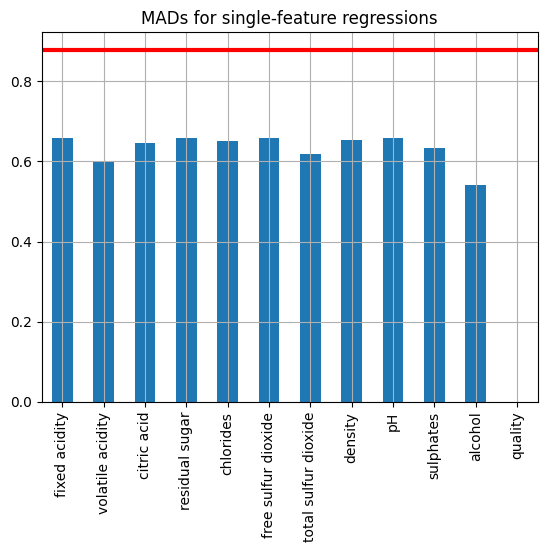wines["prediction"] = [i for i in m.var["prediction"].get_values()]
wines["quality"].hist(label="data")

wines.plot(x="quality", y="prediction", kind="scatter")
plt.show()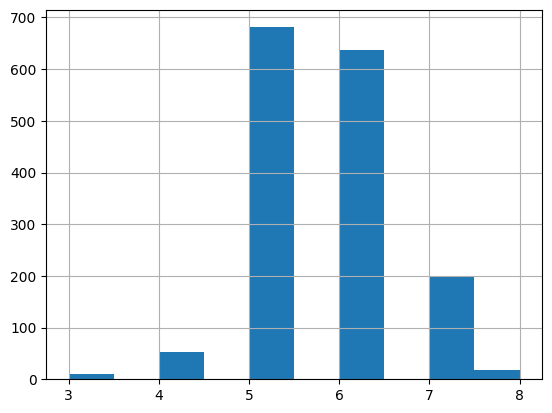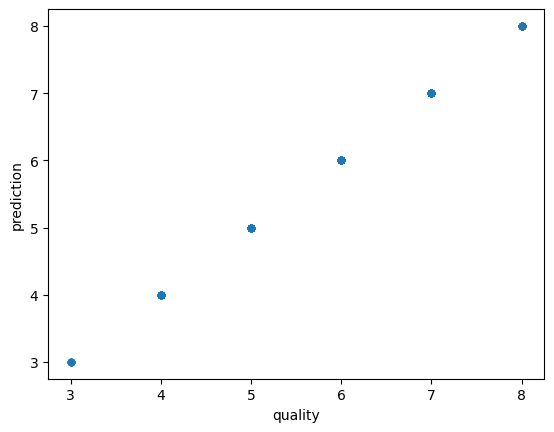## Multivariate $$L_1$$-regression#

Let us now perform a full multivariate $$L_1$$-regression on the wine dataset to predict the wine quality $$y$$ using the provided wine features. We aim to find the coefficients $$m_j$$’s and $$b$$ that minimize the mean absolute deviation (MAD) by solving the following problem:

\begin{split} \begin{align*} \text{MAD}\,(\hat{y}) = \min_{m, \, b} \quad & \frac{1}{n} \sum_{i=1}^n | y_i - \hat{y}_i| \\ \\ \text{s. t.}\quad & \hat{y}_i = \sum_{j=1}^J x_{i, j} m_j + b & \forall i = 1, \dots, n, \end{align*} \end{split}

where $$x_{i, j}$$ are values of ‘explanatory’ variables, i.e., the 11 physical and chemical characteristics of the wines. By taking care of the absolute value appearing in the objective function, this can be implemented in AMPL as an LP as follows:

%%writefile l1_fit.mod

set I;
set J;

param y{I};
param X{I, J};

var a{J};
var b;

var e_pos{I} >= 0;
var e_neg{I} >= 0;

var prediction{i in I} = sum{j in J}(a[j] * X[i, j]) + b;

s.t. prediction_error{i in I}: e_pos[i] - e_neg[i] == prediction[i] - y[i];

minimize mean_absolute_deviation: sum{i in I}(e_pos[i] + e_neg[i]) / card(I);

Writing l1_fit.mod

def l1_fit(df, y_col, x_cols):
m = AMPL()

m.set["I"] = df.index.values
m.set["J"] = x_cols

m.param["y"] = df[y_col]
m.param["X"] = df[x_cols]

m.option["solver"] = SOLVER
m.solve()

return m

m = l1_fit(
wines,
"quality",
[
"alcohol",
"volatile acidity",
"citric acid",
"sulphates",
"total sulfur dioxide",
"density",
"fixed acidity",
],
)

for k, v in m.var["a"].get_values():
print(f"{k} {v}")
print("\n")

wines["prediction"] = [i for i in m.var["prediction"].get_values()]
wines["quality"].hist(label="data")

wines.plot(x="quality", y="prediction", kind="scatter")
plt.show()

HiGHS 1.5.3: HiGHS 1.5.3: optimal solution; objective 0.4997972064
1631 simplex iterations
0 barrier iterations

alcohol 0.3424249748641086
citric acid -0.2892764146613565
density -18.50082905729104
fixed acidity 0.06381837845852499
sulphates 0.9060911918549712
total sulfur dioxide -0.002187357846776872
volatile acidity -0.9806174627416928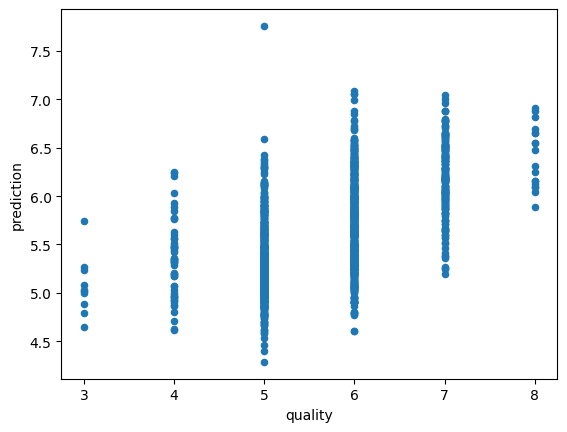## How do these models perform?#

A successful regression model would demonstrate a substantial reduction from $$\text{MAD}\,(y)$$ to $$\text{MAD}\,(\hat{y})$$. The value of $$\text{MAD}\,(y)$$ sets a benchmark for the regression. The linear regression model clearly has some capability to explain the observed deviations in wine quality. Tabulating the results of the regression using the MAD statistic we find

Regressors

none

0.683

alcohol only

0.541

all

0.500

Are these models good enough to replace human judgment of wine quality? The reader can be the judge.